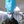0%
0%

# Python L-System

The L-System or Lindenmayer System is a mathematical system to describe the growth of plants or fractals.

Similar projects worth following
11.4k views
The L-System begins with an axiom which is a string. On this string a set of production rules are performed, which will rewrite the axiom.
This is often iterated so the output string will be fed through the production rules by the number of n.

With different rule-sets you can get very nice and interesting results from plant like sculptures to fractals like the Sierpinski triangle.

To do so I have written a small Python program.

How it works:

We choose an axiom, for example: 'A'

Now we have rules like: "A becomes AF" and "F becomes FA"

Next step is to iterate the axiom through the rules by an specific amount, the output will be fed through the rules again.

For example we iterate 3 times:

A -> AF

AF -> AFFA

AFFA -> AFFAFAAF

Our result string is now AFFAFAAF

The next step is to process the string onto our screen, for this we also have a few rules, these are:

```# F,A = move n forward# G = move n forward without drawing a line# B = move n backwards# - = turn left by angle# + = turn right by angle# [ = push position and angle# ] = pop position and angle# a,b,c,d = color 1,2,3,4# 1-4 line size (std = 1)
# other = variables```

The processing of the string works by checking char for char against the processing rules and do what they say. If we have something that doesn't fit any rules, it doesn't matter, we will just ignore it.

Processing the upper string would be lame, it will create a straight line...

Lets add a few more interesting chars to the production rules:

F -> a2FF-[c1-F+F+F]+[c1+F-F-F]

axiom: F

angle: 23

iteration: 5

Well, that looks a little bit more complicated and it is. This production rule contains nearly every possible processing rule and will create a colored tree with different sized lines.

the resulting string looks like this:

And the processed image like this:

Furthermore we can play with the rules and also have multiple rules at the same time, that can result in fancy images like the Dragoncurve:

The rules are:

X -> X+YF+

Y -> -FX-Y

axiom: FX

angle: 90

You can find a set of rules in the python program.

Another example:

Wheat \o/

### baum.py

The python program (Python 2.7)

x-python - 4.87 kB - 05/15/2016 at 19:28

• 1 × Python 2.7

• ### Wheat colored

Jana Marie05/16/2016 at 16:07 0 comments

• ### Color rule set

Jana Marie05/16/2016 at 10:42 0 comments

Colored Tree

```rules['F'] = '1aF[+2cF]1aF[-2cF]F' # tree 2axiom = 'F'angle = 25
```

• ### New Images

Jana Marie05/16/2016 at 07:53 0 comments

rules['F'] = 'F[+F]F[-F]F'

axiom = 'F'

angle = 25

rules['X'] = 'F[+X][-X]FX'

rules['F'] = 'FF'

axiom = 'X'

angle = 25

• 1
Step 1

Open the python script in the text editor of your choice.

• 2
Step 2

You will find many variables at the start of the script you can change:

Rules:

rules['X'] = 'X+YF+' # Dragon curve

rules['Y'] = '-FX-Y'

axiom = 'FX'

angle = 90

Looking like these, you can write your own or uncomment the ones already there.

iterations = 13 # number of iterations

step = 9 # step size / line length

Here you can set the number of iterations and the length of one step. Don't go too low on the step-size and too high on the iterations.

color1 = (105, 46, 26) # brown 1

color2 = (201, 146, 127) # brown 2

color3 = (101, 250, 52) # green

color4 = (255, 255, 255) # white

angleoffset = 90

size = width, height = 1920, 1080 # display with/height

Here you can set the 4 colors (currently set to tree like ones), the angle by which everything is turned and the screen-size.

# startpos = 100, height - 225

# startpos = 50, height / 2 - 50

startpos = width / 2 - 200, height / 2 +30

# startpos = 100, height / 2

# startpos = 10,10

Last one, here you can set the offset on the display

• 3
Step 3

You have to play with all that settings to get the best result. Also try different production rules. If you have/find some interestiong ones, share them please :)

Share

## Similar Projects

Project Owner Contributor

### LadyBug BEEFY: 3D printer motorized microscopeAhron Wayne

# Does this project spark your interest?

Become a member to follow this project and never miss any updates